# Shapes

As mentioned before, the shapes in this library are tiles. These classes inherit from the class `Tile`. So the three methods in the tile used to set its position (`setX`, `setY`, `setXY`) also work for them. As a tile, the position of a shape is also decided by its upper left corner.

Let's take a look at each shape in detail.

## 🔸Introduction​

### Line​

This class allows you to draw lines by setting the two endpoints.

Class

`Line` - this class is used to draw lines on a screen.

MethodExplanation
`init(x0:y0:`
`x1:y1:color:)`
Create a line.
Parameters:
-`x0`, `y0`: the coordinate of the first point.
- `x1`, `y1`: the coordinate of the second point.
- `color`: the color of the line in UInt32.

A line is also a tile. When you move a line, you set the coordinate of its upper left corner.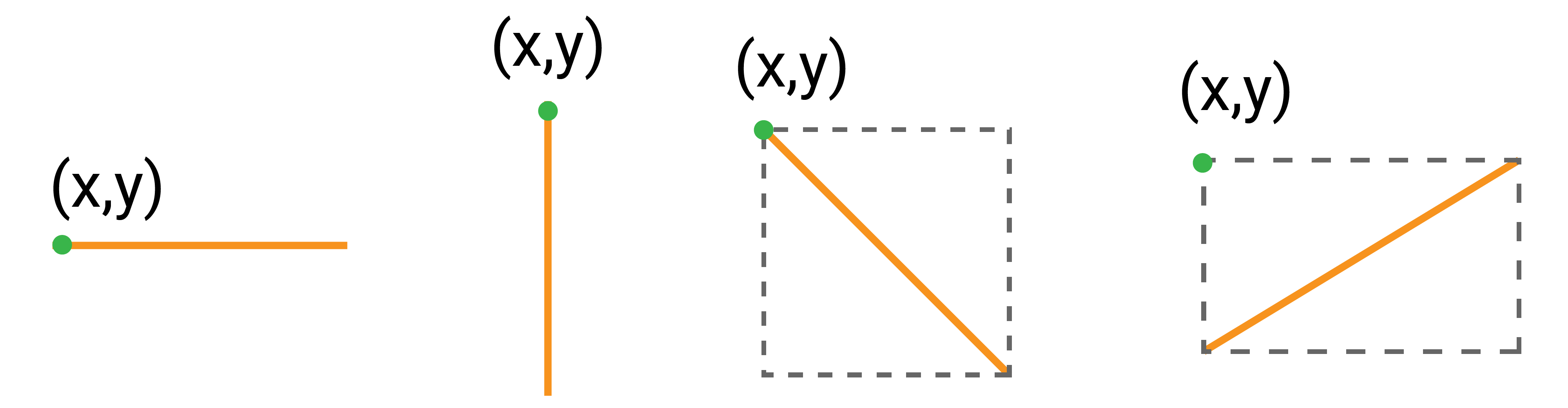Here are some examples for drawing lines:

``let line1 = Line(x0: 0, y0: 0, x1: 59, y1: 239, color: Color.red)let line2 = Line(x0: 59, y0: 239, x1: 119, y1: 0, color: Color.orange)let line3 = Line(x0: 119, y0: 0, x1: 179, y1: 239, color: Color.yellow)let line4 = Line(x0: 179, y0: 239, x1: 239, y1: 0, color: Color.green)``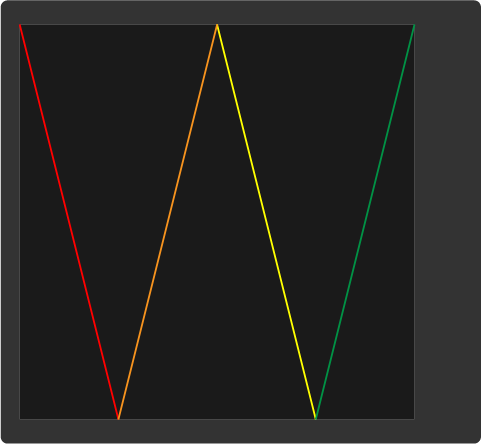### Rect​

This class allows you to draw rectangles. You can set its size, position, and color.

The upper left vertex is the starting point of a rectangle. After setting its width and height, the rest three other vertices are thus decided.

Class

`Rect` - this class is used to draw a rectangle on a screen.

MethodExplanation
`init(x:y:width:height:`
`fill:outline:stroke:)`
Create a rectangle.
Parameter:
- `x`, `y`: the coordinate of upper left corner of the rectangle.
- `height`, `width`: the height and width of the rectangle to decide its size.
- `fill`: the color of the rectangle.
- `outline`: the color of the outline.
- `stroke`: the width of the outline, 1 by default. It takes up the rectangle's inner area and doesn't change the size of the entire shape.
`fill(color:)`Fill the inner area of the rectangle with the specified color.
`setOutline(color:)`Set the color of the stroke.

Let’s see how you create rectangles:

``let rect1 = Rect(x: 0, y: 0, width: 65, height: 50, fill: Color.yellow, outline: Color.orange, stroke: 2)let rect2 = Rect(x: 10, y: 150, width: 40, height: 40, fill: Color.yellow)let rect3 = Rect(x: 100, y: 0, width: 65, height: 50, outline: Color.orange)``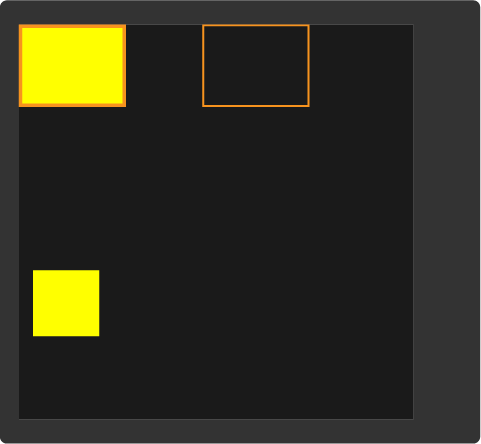### RoundRect​

This class allows you to draw rounded rectangles. You can regard it as a combination of 4 lines and 4 quarter circles.

Class

`RoundRect` - this class is used to draw a rounded rectangle on a screen.

MethodExplanation
`init(x:y:`
`width:height:`
`radius:fill:`
`outline:stroke:)`
Create a rounded rectangle.
Parameter:
- `x`, `y`: the coordinate of upper left corner of this tile.
- `height`, `width`: the height and width of the rounded rectangle.
- `radius:` the radius of the rounded corner which is a quarter circle.
- `fill`: the color of the shape.
- `outline`: the color of the outline.
- `stroke`: the width of the outline, 1 by default. It takes up the rounded rectangle's inner area and doesn't change the size of the shape.
`fill(color:)`Fill the rounded rectangle with the specified color.
`outline(color:)`Set the color of its outline.

In the image below, the orange area is a rounded rectangle. The dashed line marks all pixels for this tile. The coordinate you set when creating a rectangle is the upper left vertex of the tile, marked with a green dot.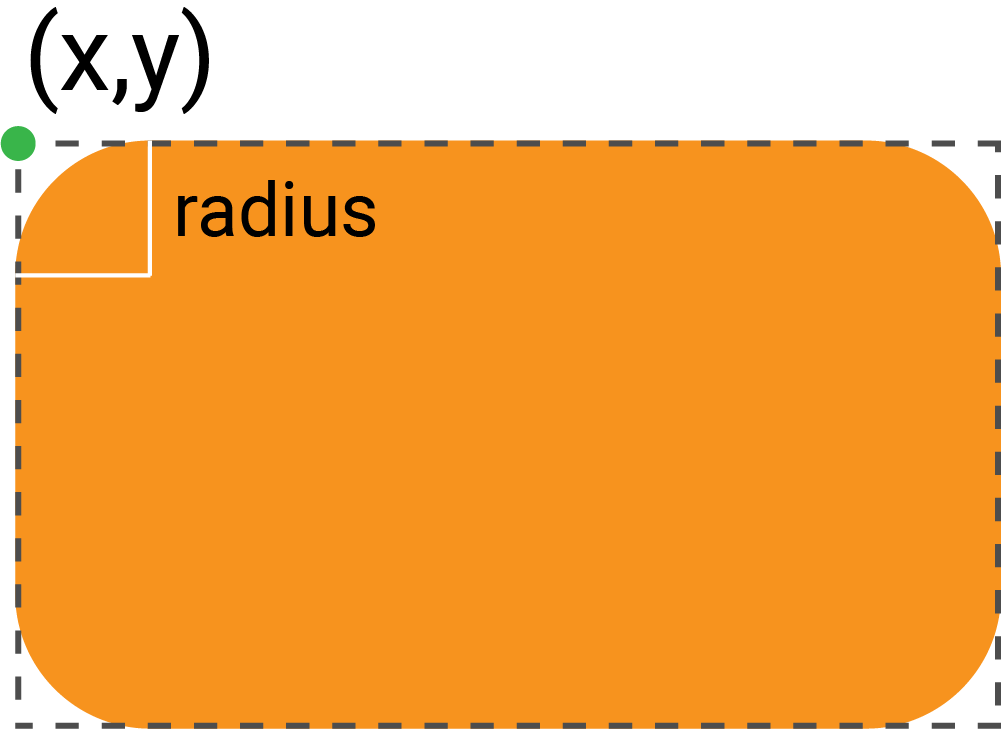Let’s look at some examples:

``let roundRect1 = RoundRect(x: 15, y: 20, width: 55, height: 70, radius: 5, fill: Color.magenta, outline: Color.white, stroke: 3)let roundRect2 = RoundRect(x: 100, y: 50, width: 70, height: 70, radius: 5, fill: Color.magenta)let roundRect3 = RoundRect(x: 40, y: 40, width: 55, height: 70, radius: 5, outline: Color.white, stroke: 2)``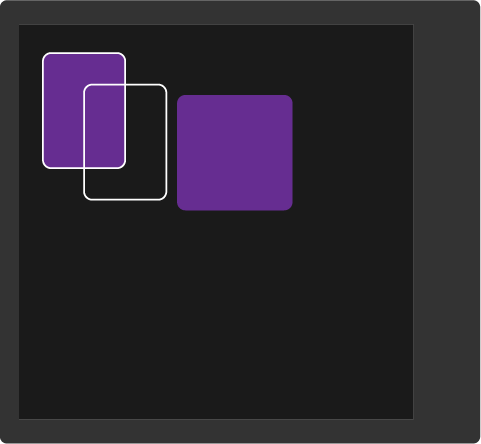### Circle​

This class allows you to draw circles on a screen.

As you create a circle, its position is decided by its center. To move it, you set the coordinate of the upper left corner of the tile.

Class

`Circle` - this class is used to draw a circle on a screen.

MethodExplanation
`init(x:y:radius:fill:`
`outline:stroke:)`
Create a circle.
Parameters:
- `x`, `y`: the position of the circle by setting the center.
- `radius`: the radius of the circle.
- `fill`: the color of the shape.
- `outline`: the color of the outline.
- `stroke`: the width of the outline, 1 by default. It takes up the inner area and does not change the size of the shape.
`fill(color:)`Set the color of the circle.
`outline(color:)`Set the color of its outline.

Let’s create some circles:

``let circle1 = Circle(x: 50, y: 50, radius: 50, fill: Color.green, outline: Color.yellow, stroke: 2)let circle2 = Circle(x: 30, y: 80, radius: 20, fill: Color.white)let circle3 = Circle(x: 150, y: 150, radius: 50, outline: Color.yellow)``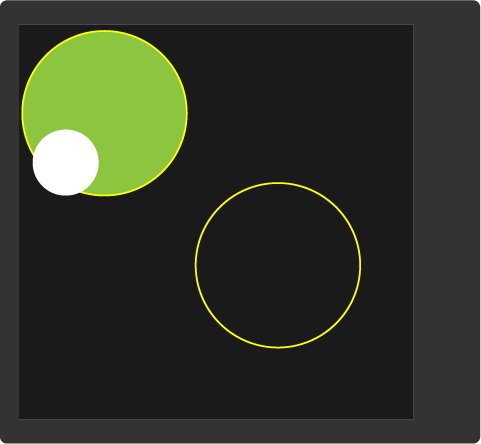### Triangle​

You can use it to create triangles. 3 vertices are needed to decide a triangle.

Class

`Triangle` - this class is used to draw a triangle on a screen.

MethodExplanation
`init(x0:y0:`
`x1:y1:x2:y2:`
`fill:outline:)`
Create a rounded rectangle.
Parameters:
- `x0`, `y0`, `x1`, `y1`, `x2`, `y2`: the coordinates of 3 vertices.
- `fill`: the color of the triangle.
- `outline`: the color of the outline.
`fill(color:)`Change the color of the triangle.

Let’s draw triangles:

``let triangle1 = Triangle(x0: 0, y0: 0, x1: 0, y1: 100, x2: 50, y2: 50, fill: Color.purple, outline: Color.red)let triangle2 = Triangle(x0: 100, y0: 30, x1: 39, y1: 135, x2: 161, y2: 135, fill: Color.lime, outline: Color.white)``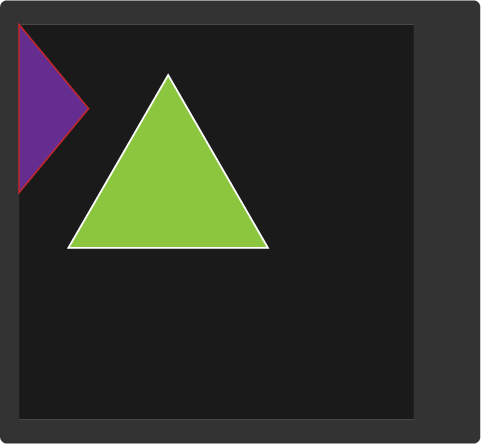### Polygon​

It allows you to draw a random shape. You can set any number of points. Then they will be connected in order and create a closed shape.

Class

`Polygon` - this class is used to draw a polygon on a screen.

MethodExplanation
`init(_:outline:)`Create a polygon.
Parameters:
- `points`: an array of coordinates of all vertices.
- `outline`: the color of the stroke.

Let’s create some polygons:

``let points1 = [(100,50), (52,85), (71,140), (129,140), (148,85)]let pentagon = Polygon(points, outline: Color.red)let points2 = [(170,115), (130,115), (110,150), (130,185), (170,185), (190,150)]let hexagon = Polygon(points2, outline: Color.green)``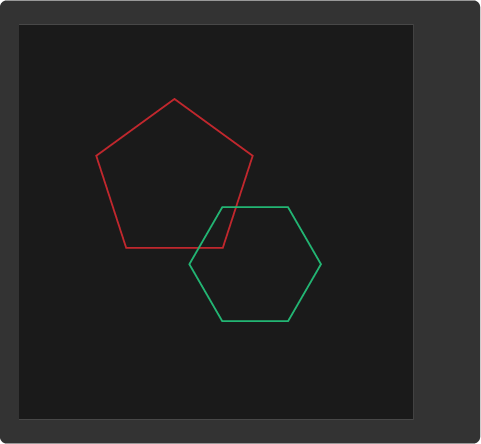You could get the coordinates of regular polygons using this online tool. You could also create a random shape with this online geometry tool and get the coordinates.

## 🔸Project​

### 1. Simple shapes drawing​

The first project gets a quick view of all shapes.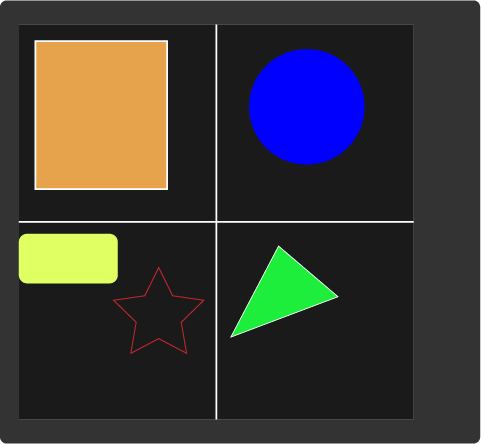``// Import the SwiftIO library to set SPI communication and MadBoard to use pin id.import SwiftIOimport MadBoard// Import the driver for the screen and graphical library for display.import ST7789import MadDisplay// Initialize the pins for the screen.let spi = SPI(Id.SPI0, speed: 30_000_000)let cs = DigitalOut(Id.D9)let dc = DigitalOut(Id.D10)let rst = DigitalOut(Id.D14)let bl = DigitalOut(Id.D2)// Initialize the screen with the pins above.let screen = ST7789(spi: spi, cs: cs, dc: dc, rst: rst, bl: bl, rotation: .angle90)let display = MadDisplay(screen: screen)let group = Group()// Create two lines and append them to the group.let line1 = Line(x0: 0, y0: 119, x1: 240, y1: 119, color: Color.white)let line2 = Line(x0: 119, y0: 0, x1: 119, y1: 240, color: Color.white)group.append(line1)group.append(line2)// Create a rectangle and add it to the group.let rect = Rect(x: 10, y: 10, width: 80, height: 90, fill: Color.orange, outline: Color.white)group.append(rect)// Create a circle and add it to the group.let circle = Circle(x: 175, y: 50, radius: 35, fill: Color.blue)group.append(circle)// Create a rounded rectangle and add it to the group.let roundRect = RoundRect(x: 0, y: 130, width: 60, height: 30, radius: 5, fill: Color.yellow)group.append(roundRect)// Create a triangle and add it to the group.let triangle = Triangle(x0: 130, y0: 200, x1: 160, y1: 140, x2: 200, y2: 180, fill: Color.lime, outline: Color.white)group.append(triangle)// Set the vertices of a star and use them to create a star.let points = [(85,150), (92,172), (115,172), (95,186), (105,210), (85,194), (65,210), (75,186), (55,172), (78,172)]let polygon = Polygon(points, outline: Color.red)group.append(polygon)// Display all shapes on the screen.display.update(group)while true {    sleep(ms: 1000)}``

### 2. Shape animation​

In this project, you'll use some shapes above to make some patterns to create a simple animation.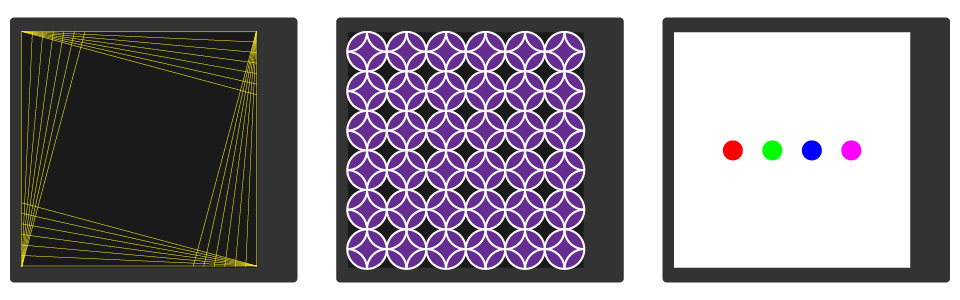Example code

``// import the SwiftIO library to set SPI communication and MadBoard to use pin id.import SwiftIOimport MadBoard// Import the driver for the screen and graphical library for display.import ST7789import MadDisplay// Initialize the pins for the screen.let spi = SPI(Id.SPI0, speed: 30_000_000)let cs = DigitalOut(Id.D9)let dc = DigitalOut(Id.D10)let rst = DigitalOut(Id.D14)let bl = DigitalOut(Id.D2)// Initialize the screen with the pins above.let screen = ST7789(spi: spi, cs: cs, dc: dc, rst: rst, bl: bl, rotation: .angle90)// Create an instance using the screen for dispay later.let display = MadDisplay(screen: screen)let group = Group()// Draw yellow lines on the screen. for y in stride(from: 0, to: 80, by: 10) {    drawLine(x0: 0, y0: 0, x1: 239, y1: y, color: Color.yellow)}for x in stride(from: 239, to: 160, by: -10) {    drawLine(x0: 239, y0: 0, x1: x, y1: 239, color: Color.yellow)}for y in stride(from: 239, to: 160, by: -10) {    drawLine(x0: 239, y0: 239, x1: 0, y1: y, color: Color.yellow)}for x in stride(from: 0, to: 80, by: 10) {    drawLine(x0: 0, y0: 239, x1: x, y1: 0, color: Color.yellow)}// Update the screen each time you draw a line.func drawLine(x0: Int, y0: Int, x1: Int, y1: Int, color: UInt32) {    let line = Line(x0: x0, y0: y0, x1: x1, y1: y1, color: color)    group.append(line)    display.update(group)}// Draw a black rectangle as backgroud to hide the lines.// It will move gradually from the right.let blackBg = Rect(x: 240, y: 0, width: 240, height: 240, fill: Color.black)group.append(blackBg)for x in stride(from: 240, through: 0, by: -20) {    blackBg.setX(x)    display.update(group)}// Fill the screen with circles.for y in stride(from: 20, to: 280, by: 40) {    for x in stride(from: 20, to: 280, by: 40) {        let circle = Circle(x: x, y: y, radius: 20, fill: Color.purple, outline: Color.white, stroke: 2)        group.append(circle)    }}display.update(group)for y in stride(from: 0, to: 280, by: 40) {    for x in stride(from: 0, to: 280, by: 40) {        let circle = Circle(x: x, y: y, radius: 20, outline: Color.white, stroke: 2)        group.append(circle)    }}display.update(group)sleep(ms: 1000)// Remove all tiles in the group to clear the screen.while group.getLength() > 0 {    group.pop()}display.update(group)// Draw a white rectangle used as background later.let whiteBg = Rect(x: 0, y: 0, width: 240, height: 240, fill: Color.white)group.append(whiteBg)display.update(group)sleep(ms: 1000)// Create four circles used for animation later.let circle0 = Circle(x: 10, y: 119, radius: 10, fill: Color.red)let circle1 = Circle(x: 50, y: 119, radius: 10, fill: Color.lime)let circle2 = Circle(x: 90, y: 119, radius: 10, fill: Color.blue)let circle3 = Circle(x: 130, y: 119, radius: 10, fill: Color.magenta)let circles = [circle0, circle1, circle2, circle3]// Create a subgroup for the circles.let circleGroup = Group()group.append(circleGroup)while true {    // Add the circle to the circleGroup and move the circleGroup to display it on the middle of the screen.    // You will see the circles appear one after another on the screen.    for i in 0..<circles.count {        circleGroup.append(circles[i])        circleGroup.setX(109 - i * 20)        display.update(group)        sleep(ms: 1000)    }    // Remove the circles from the circleGroup and display the circleGroup on the middle of the screen.    // You will see circles disappear one by one.    for i in (0..<circles.count).reversed() {        circleGroup.remove(circles[i])        circleGroup.setX(129 - i * 20)        display.update(group)        sleep(ms: 1000)    }}``